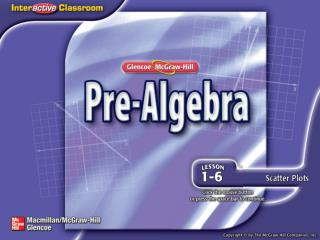Download PresentationSplash Screen

# Splash Screen - PowerPoint PPT PresentationDownload Presentation## Splash Screen

- - - - - - - - - - - - - - - - - - - - - - - - - - - E N D - - - - - - - - - - - - - - - - - - - - - - - - - - -
##### Presentation Transcript

1. Splash Screen

2. A B C D Which expression can be used to represent fourteen less than twice the width? A. 14 – 2 + w B. 14 – 2w C. 2w + 14 D. 2w – 14 Which expression can be used to find the number of quarts in any number of gallons? A. B.q = 4g C.4q = g D.16q = g 5-Minute Check 1

3. You have already graphed ordered pairs and relations on a coordinate system. (Lesson 1–4) • Construct scatter plots. • Analyze trends in scatter plots. Then/Now

4. scatter plot Vocabulary

5. Construct a Scatter Plot BREADThe table shows the average cost of a loaf of bread from 1920–2000. Make a scatter plot of the data. Let the horizontal axis, or x-axis, represent the year.Let the vertical axis, or y-axis, represent the cost. Example 1

6. Construct a Scatter Plot BREADThe table shows the average cost of a loaf of bread from 1920–2000. Make a scatter plot of the data. Answer: Graph the ordered pairs (year, cost). Example 1

7. A B C D A. B. C.D. BIRTH STATISTICSThe table shows the number of babies born at Central Hospital during the past eight months. Make a scatter plot of the data. Example 1

8. Example: The hours you study and your test average have a positive relationship (as your hours go up, so do your scores) Example: The minutes a candle is burned and the height of the candle have a negative relationship (the longer you burn, the shorter the candle) Example: The number of slices of pizza you eat and your shoe size (the is no obvious relation to these two pieces of data) Concept

9. Interpret Scatter Plots Determine whether the scatter plot of the heights of basketball players and number of rebounds shows a positive, negative, or no relationship. Explain your answer. As height increases, the number of rebounds increases. Answer: positive relationship Example 2

10. .1 Determine whether the scatter plot of the weight of a car and its miles per gallon shows a positive, negative, or no relationship. Explain your answer. As the weight of the car (x-value) increases the miles per gallon (y-value) decreases. Answer: negative relationship

11. A B C D Determine whether a scatter plot of the outside temperatures and the corresponding heating bill might show a positive, negative, or no relationship. Explain your answer. A. As the outside temperature increases, the heating bill will decrease. This is a negative relationship. B. As the outside temperature increases, the heating bill will decrease. This is a positive relationship. C. As the outside temperature increases, the heating bill will increase. This is a negative relationship. D. Outside temperature and heating bill have no relationship. Example 2

12. Analyze Scatter Plots A. TEMPERATURE The table shows temperatures in degrees Celsius and the corresponding temperatures in degrees Fahrenheit. Make a scatter plot of the data. Let the horizontal axis represent degrees Celsius and the vertical axis represent degrees Fahrenheit. Graph the data. Example 3

13. Analyze Scatter Plots B. Draw a conclusion about the type of relationship the data shows. Explain. Answer: A positive relationship is shown. As °C increase, so do °F. Example 3

14. Analyze Scatter Plots C. Predict the Fahrenheit temperature for 35°C. By looking at the pattern on the graph, we can predict that the Fahrenheit temperature corresponding to 35°C would be about 95 degrees. Answer: 95°F Example 3

15. A B C D A.B. C.D. A. STUDY SKILLS The table shows hours spent studying for a test and the corresponding test score. Make a scatter plot of the data. Example 3

16. A B C D B. STUDY SKILLS The table shows hours spent studying for a test and the corresponding test score. Does the scatter plot show a relationship between hours studied and a student’s test score? A. Yes, a positive relationship exists. B. Yes, a negative relationship exists. C. No, no relationship exists. D. none of the above Example 3

17. A B C D C. STUDY SKILLS The table shows hours spent studying for a test and the corresponding test score. Predict the test score for a student who spends 7 hours studying. A. between 65 and 70 B. between 75 and 80 C. between 95 and 100 D. between 105 and 110 Example 3

18. End of the Lesson